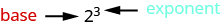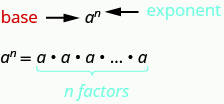## Identifying Expressions and Equations

### Learning Outcomes

• Identify and write mathematical expressions using symbols and words
• Identify and write mathematical equations using symbols and words
• Identify the difference between an expression and an equation
• Use exponential notation to express repeated multiplication
• Write an exponential expression in expanded form

## Identify Expressions and Equations

What is the difference in English between a phrase and a sentence? A phrase expresses a single thought that is incomplete by itself, but a sentence makes a complete statement. “Running very fast” is a phrase, but “The football player was running very fast” is a sentence. A sentence has a subject and a verb.

In algebra, we have expressions and equations. An expression is like a phrase. Here are some examples of expressions and how they relate to word phrases:

Expression Words Phrase
$3+5$ $3\text{ plus }5$ the sum of three and five
$n - 1$ $n$ minus one the difference of $n$ and one
$6\cdot 7$ $6\text{ times }7$ the product of six and seven
$\frac{x}{y}$ $x$ divided by $y$ the quotient of $x$ and $y$

Notice that the phrases do not form a complete sentence because the phrase does not have a verb. An equation is two expressions linked with an equal sign. When you read the words the symbols represent in an equation, you have a complete sentence in English. The equal sign gives the verb. Here are some examples of equations:

Equation Sentence
$3+5=8$ The sum of three and five is equal to eight.
$n - 1=14$ $n$ minus one equals fourteen.
$6\cdot 7=42$ The product of six and seven is equal to forty-two.
$x=53$ $x$ is equal to fifty-three.
$y+9=2y - 3$ $y$ plus nine is equal to two $y$ minus three.

### Expressions and Equations

An expression is a number, a variable, or a combination of numbers and variables and operation symbols.
An equation is made up of two expressions connected by an equal sign.

### example

Determine if each is an expression or an equation:

1. $16 - 6=10$
2. $4\cdot 2+1$
3. $x\div 25$
4. $y+8=40$

Solution

 1. $16 - 6=10$ This is an equation—two expressions are connected with an equal sign. 2. $4\cdot 2+1$ This is an expression—no equal sign. 3. $x\div 25$ This is an expression—no equal sign. 4. $y+8=40$ This is an equation—two expressions are connected with an equal sign.

## Simplify Expressions with Exponents

You have simplified many expressions so far using the four main mathematical operations. To simplify a numerical expression means to do all the math possible. For example, to simplify $4\cdot 2+1$ we’d first multiply $4\cdot 2$ to get $8$ and then add the $1$ to get $9$. A good habit to develop is to work down the page, writing each step of the process below the previous step. The example just described would look like this:

$4\cdot 2+1$
$8+1$
$9$

However, there are other mathematical notations used to simplify the numbers we are working with. Suppose we have the expression $2\cdot 2\cdot 2\cdot 2\cdot 2\cdot 2\cdot 2\cdot 2\cdot 2$. We could write this more compactly using exponential notation. Exponential notation is used in algebra to represent a quantity multiplied by itself several times. We write $2\cdot 2\cdot 2$ as ${2}^{3}$ and $2\cdot 2\cdot 2\cdot 2\cdot 2\cdot 2\cdot 2\cdot 2\cdot 2$ as ${2}^{9}$. In expressions such as ${2}^{3}$, the $2$ is called the base and the $3$ is called the exponent. The exponent tells us how many factors of the base we have to multiply.$\text{means multiply three factors of 2}$
We say ${2}^{3}$ is in exponential notation and $2\cdot 2\cdot 2$ is in expanded notation.

### Exponential Notation

For any expression ${a}^{n},a$ is a factor multiplied by itself $n$ times if $n$ is a positive integer.

${a}^{n}\text{ means multiply }n\text{ factors of }a$The expression ${a}^{n}$ is read $a$ to the ${n}^{th}$ power.

For powers of $n=2$ and $n=3$, we have special names.

$a^2$ is read as “$a$ squared”

$a^3$ is read as “$a$ cubed”

The table below lists some examples of expressions written in exponential notation.

Exponential Notation In Words
${7}^{2}$ $7$ to the second power, or $7$ squared
${5}^{3}$ $5$ to the third power, or $5$ cubed
${9}^{4}$ $9$ to the fourth power
${12}^{5}$ $12$ to the fifth power

### example

Write each expression in exponential form:

1. $16\cdot 16\cdot 16\cdot 16\cdot 16\cdot 16\cdot 16$
2. $\text{9}\cdot \text{9}\cdot \text{9}\cdot \text{9}\cdot \text{9}$
3. $x\cdot x\cdot x\cdot x$
4. $a\cdot a\cdot a\cdot a\cdot a\cdot a\cdot a\cdot a$

### try it

In the video below we show more examples of how to write an expression of repeated multiplication in exponential form.

### example

Write each exponential expression in expanded form:

1. ${8}^{6}$
2. ${x}^{5}$

### try it

To simplify an exponential expression without using a calculator, we write it in expanded form and then multiply the factors.

### example

Simplify: ${3}^{4}$

## Contribute!

Did you have an idea for improving this content? We’d love your input.